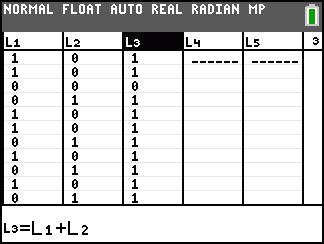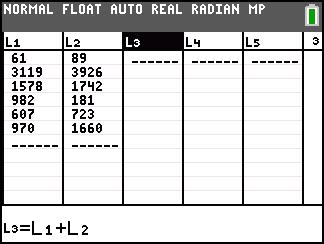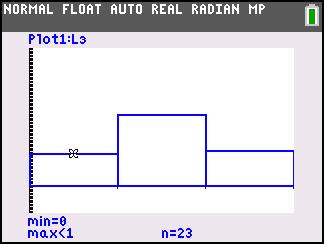# Activities

••• ##### Subject Area

• Math: Statistics: Probability and Random Variables

• ##### Author9-12

45 Minutes

• ##### Device
• TI-83 Plus Family
• TI-84 Plus
• TI-84 Plus Silver Edition
•TI-84 Plus C Silver Edition
•TI-84 Plus CE
• ##### Report an Issue

Independence is the Word#### Activity Overview

Students use a simulation to find the experimental probability of independent events.

#### Key Steps

•Students will simulate two independent events, two tosses of one coin, using a lists and the randInt command. They will use lists L1and L2 to represent the tossing of the first coin and the second coin respectively. Then students will use list L3 to sum each row, where each row represents one trial.

•In the second part of the activity, students will investigate conditional probability. The questions on the worksheet will direct them towards a better understanding of how conditional probabilities are different from independent probabilities are how the formula is used to compute a probability.

•Discuss the material from the activity pages with students as needed.